# Decline Curve Analysis

Jump to: navigation, search

## Brief

Decline Curve Analysis (DCA) is an empirical method for rate decline analysis and rate forecasting published by Arps in 1945 .

DCA is applied for Wells and Reservoirs production forecasting.

E&P Portal has DCA available as one of it's engineering tools.

DCA in the E&P Portal

## Math & Physics

Note  Rate Cumulative
Hyperbolic decline
0 < b < 1, b > 1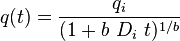$q(t) = \frac{q_i}{(1+b\ D_i\ t)^{1/b}}$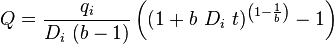$Q = \frac{q_i}{D_i\ (b-1)} \left ( (1+b\ D_i\ t)^ \left ( 1-\frac{1}{b} \right ) -1 \right )$
Exponential decline
b = 0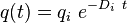$q(t) = {q_i}\ e^{-D_i\ t}$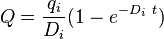$Q = \frac{q_i}{D_i}(1-e^{-D_i\ t})$
Harmonic decline
b = 1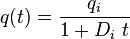$q(t) = \frac{q_i}{1+D_i\ t}$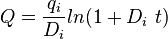$Q = \frac{q_i}{D_i} ln{(1+D_i\ t)}$

## Discussion

If one has a need to convert decline factor Di to the actual annual decline in %: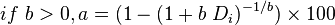$if\ b > 0, a = (1 - (1 + b\ D_i)^{- 1 / b}) \times 100$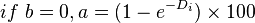$if\ b = 0, a = (1 - e^{-D_i}) \times 100$

## Workflow

1. Upload the data required
2. Open the DCA tool here
3. For a single well
1. Select a well in a filter
2. Input the initial rate,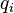$q_i$
3. Drag the Navigation Point to match the actual data
4. Correct the model parameters manually if needed
5. Check the difference in cumulative production of actual cure vs decline curve
6. Check the Rate vs Cumulaive Plot in linear and log scale
7. Save the decline curve model
8. Move to the next well
4. For a multiple wells
1. Select the wells in the filter
2. DCA tool will automatically normalize the wells data to zero time and then averages it
3. Input the initial rate,$q_i$
4. Drag the Navigation Point to match the actual data
5. Correct the model parameters manually if needed
6. Check the difference in cumulative production of average cure vs decline curve
7. Check the Rate vs Cumulaive Plot in linear and log scale
8. Export the type curve if needed

### Nomenclature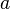$a$ = annual decline, %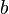$b$ = decline curve parametr, dimensionless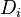$D_i$ = decline factor per time t, dimensionless$q_i$ = initial rate, any rate units applies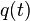$q(t)$ = rate at time t, any rate units applies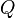$Q$ = cumulatve rate at time t, any rate units applies$t$ = forecast time, days